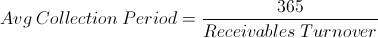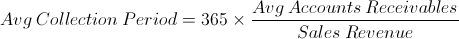# Average Collection PeriodThe average collection period formula is the number of days in a period divided by the receivables turnover ratio.

The numerator of the average collection period formula shown at the top of the page is 365 days. For many situations, an annual review of the average collection period is considered. However, if the receivables turnover is evaluated for a different time period, then the numerator should reflect this same time period.

For example, if the receivables turnover for one year is 8, then the average collection period would be 45.63 days. If the period considered is instead for 180 days with a receivables turnover of 4.29, then the average collection period would be 41.96 days. By the nature of the formula, a company will have a lower receivables turnover when a shorter time period is considered due to having a larger portion of its revenues awaiting receipt in the short run.

## How is the Average Collection Period Formula Derived?

In order to understand the concept of the average collection period formula, one must first look at the accounts receivables turnover formula ofThe average collection period formula can be rewritten as the numerator, 365 days, times the inverse of the denominator. This would result in the formulaThe 2nd portion of this formula is essentially the % of sales that is awaiting payment. The % of sales awaiting payment is then used as the % of time awaiting payment throughout the period. From here, the % of time awaiting payment is converted into actual days by multiplying by 365.

It is important to consider that a company that has seasonal sales will affect the outcome when using this formula. For example, a company that sales mostly at the beginning of the period may show none or very little payments awaiting receipt. Also, a company who sales mostly towards the end of the period may show a very high amount of receivables. Alterations of the formula may be required to adjust for each company.

New to Finance?